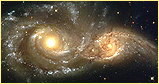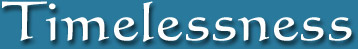Home  |  Part One  |  Part Two  |  Part Three  |  Part Four  |  Part Five  |  Contents  |  Backward  |  Forward

 Zero is powerful because it is infinitys twin. They are equal and opposite, yin and yang. They are equally paradoxical and troubling. The biggest questions in science and religion are about nothingness and eternity, the void and the infinite, zero and infinity. The clashes over zero were the battles that shook the foundations of philosophy, of science, of mathematics, and of religion. Underneath every revolution lay a zero  and an infinity.  Charles Seife

"I am at two with nature."
Woody Allen

Excerpt from Chapter22
From The Book:
Everything Forever:
Learning to See Timelessness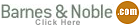"one could say that through the human being, the universe is making a mirror to observe itself."
David Bohm and Basil Hiley

"The universe can be best pictured, though still very imperfectly and inadequately, as consisting of pure thought, the thought of what we must describe as a mathematical thinker."
Sir James Jeans

Excerpt From Chapter 22

#### The Infinite yet fully Definite Plane of Symmetry Math

The nature of infinity is very much an unresolved mystery in both physics and mathematics, but there are a few tolerated infinities, such as electrons and black holes. And there are infinite series equations which mathematicians say are defined because they express Convergence. For example, .999... is said to be equal to 1, even if the universes fastest computer would never be able to appreciate the .999as a single definite value.

Other examples of convergence include:

The sequence:  1/1, 1/2, 1/3, 1/4 ...  is converging toward 0.

The equation:  4 - 2 - 1 - 1/2 - 1/4 - 1/8 - ...  is converging to 0 also.

The equation:  1/2 + 1/4 + 1/8 + 1/16 ...  is converging toward a limit of one.

And the equation:  1 + 1/2 + 1/4 + 1/8 + 1/16 + ...  is converging toward 2

Obviously (1 + 1 + 1 + ...) is not a convergent series, it has an increasing value, so there is never a completed sum. There is no convergence. In ordinary math we think of the set of positive or negative numbers as continuous and indefinite.

In symmetry math there is one ultimate value which contains all other numbers and on either side of Omega Zero other values decrease. As we count into ever larger numerals, the symmetry value decreases toward an infinitely small value. Consequently, the values of larger numerals converge toward two outer extreme points. This clearly visible convergence allows positive infinity and negative infinity to have definite values. So they can be represented as two numbers. Consider what a relief this model is to the anxiety of indefiniteness in ordinary math.

Unlike our present finite system of values, which definitely does not consider positive or negative infinity to be a number, in symmetry math we can define two final numbers on either side of zero. In symmetry math, if we add ones endlessly (1+1+1+ ...), there is still always a next greater numeral, but the value of the sum is decreasing and converging toward a point of infinitely small value. That point is a last number. I call the convergent sum for positive numbers Proto, which means first in time. Proto is a numeric representing positive infinity, here written +. I call the ultimate negative number Elea, the sum of all negative numbers. Obviously I derived the names from the proton and electron.

In treating a positive and a negative infinity as numbers, we then can write this simple equation:

+  +  -∞ = = 0

All of symmetry math is defined and whole, bounded by extremes, just as pattern space is defined and whole. The symmetry plane is infinite but bounded. As a result, we can begin from Proto or Elea and count toward zero.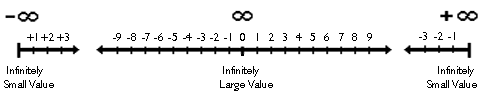Figure 22.1:  In symmetry math the whole mathematical
number line is a spectrum of infinities extending out
from a whole infinity toward two half infinities.

In ordinary math the nothing of zero cannot relate to positive or negative infinity in the way convergence allows the extreme values of this symmetry mathematical plane to relate. Unlike ordinary math, in symmetry math we can say both sides of the plane are perfectly balanced around zero and not balanced around any other number, simply because Proto and Elea are definite and absolute values. They are definable as numbers, where in ordinary math there arent ultimate positive and negative numbers, since we can never reach the end value of a non-converging series.

We can now reconsider the issue of there being three incompatible answers when summing all reals or integers. If we keep in mind that in symmetry math we have boundaries, zero in the middle, and Proto and Elea on opposite ends, we then again consider the equation:

(1 + 0) + (2 + (-1)) + (3 + (-2)) + (4 + (-3)) + ...

Since positive infinity is a number in symmetry math, we can count away from positive infinity toward zero, and so we can consider an end to this equation. As shown below, now the equation ends definitively with the same displacement that it began with, and since the equation is a sum of all numbers, the equation ends by also summing the number Elea into the final sum, causing the equation to equal zero, as shown below: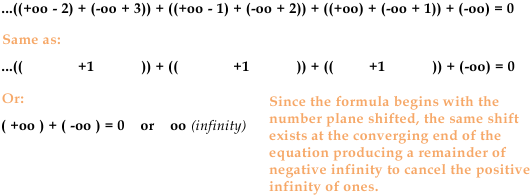Figure 22.2:  Notice that Proto can be subtracted from or reduced, but not added to. Elea can be added to but
not subtracted from. Also note that in symmetry math the addition of -∞ and +∞ is actually a combining of
both sets into an everything, rather than a cancellation of positive and negative which creates nothing.

Math and Order

One of the surprising and elegant features of symmetry math is that although the two smallest numbers, Proto and Elea, are points of infinitely small value, each number still represents half of the whole. What do I mean by half of the whole? Where the symmetry value of the number one includes all numbers except (-1), the symmetry value of Proto equals all numbers except all the negative numbers. All the negative numbers must be made separate, just as grouping order separates the game pieces of checkers. All we can do is remove all the negative numbers, or all the positive numbers, from the fullness of zero, which makes Proto and Elea the two smallest possible values in this system. Consequently we dont have a value of nothing in this system. Proto and Elea are as small as any values can become. So even though Proto contains the infinity of all positive numbers, relative to all other values, it is infinitely small.

Obviously the infinitely small value of Proto (and Elea) can be related to the infinitely small size of the Alpha state of the big bang. Just as spacetime collapses in our past to a smallest possible size, Proto and Elea are equivocally the smallest possible values in symmetry math. Then just as the Omega zero of symmetry math is infinitely large and contains all numbers, the expanding universe ultimately becomes a space that extends infinitely in all directions. Symmetry math is essentially a mathematical model of the Universe.

Symmetry math provides a great deal of insight into the properties of the Alpha state from which time originated. Proto is all positive numbers grouped apart and unified into a positive singularity. Proto is a positive oneness, an ultimate one that is positive, like the 1 byte of computers. Some of Alphas properties such as "uniformity" are similar to Omegas properties. The greatest difference is energy content. Since the very nature of being positive or negative is imbalance, the extreme positive of Alpha is naturally a very energetic state, a probabilistic energy all aimed at moving toward balance. Some big bang theorists describe time originating from a false vacuum, which is an empty space that for some reason isn't stable.

Symmetry Math and Space

In the same way that we live caught between two kinds of order, in terms of space we live in between a three dimensional (3D) space and a four dimensional space (4D), so we live in a fractional 3.x space. We might guess that we live in a 3.7304572 dimensional universe, that is expanding and so moving ever nearer to the complete 4D universe. Further, I believe a negative curvature is properly understood as being identical to an expanding space, and a positive curvature is identical to gravitational contraction (both are necessarily temporal). A negative curvature is time moving forward. A positive curvature is time moving backwards.

Relative to our expanded 3.x space, the Alpha space in our past, which is only a 3D space, is infinitely curved (and hot). Even though Alpha is a simple flat and empty infinitely extended space, that space is the smallest space possible and appears infinitely small relative to our much larger 3.x expanded space. So for example, a contracting universe (big crunch) could only reduce to a 3D space which we perceive from within spacetime to be a point. This explains why some physicists see a vacuum at the beginning of time while others see an infinitely dense singularity. They are the same state. Inversely, an expanding space ultimately becomes a 4D space (after a big rip type of ending), which is an absolutely full 4D space, meaning it contains all possible curvatures, or all 3.x fractional states (same as superposition of all possible universes).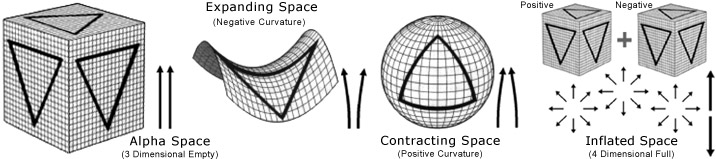An expanding universe is the result of a single 3D (positive) space colliding with a single 3D (negative) space, i.e., Proto and Elea, which causes the big bang. A summing of the two spaces results in a perfectly flat, fully inflated 4D space, i.e., the absolutely stable true vacuum, which is the absolute zero of all physics (a neutral space). Brett McInnes (2002 big smash paper) and Robert Caldwell (2002 big rip paper) have both referred to the end of time as the "final" and "ultimate singularity". Time ends as the ultimate 4D singularity. And this 4D singularity is the native state of reality, i.e., the default setting. It is the nothing that is everything. The two 3D spaces (positive and a negative) are inevitable components of a 4D space, two perfect halves of the 4D whole. A Euclidean 3D space can only expand, contracting toward a 2D or 1D space violates conservation of the eternal 4D whole (although we can understand the components of a 3D space as built from lesser dimensions. I prefer Rob Bryanton's model where a one dimensional line, and a two dimensional split, and a third dimensional fold, produce a three dimensional space that is seen as a point from higher dimensions. Note that in Bryanton's model we can more clearly recognize the full space (Omega) in our future to actually be 6 dimensional, 3D + 3D = 6D, i.e., time equals 3 dimensions of space).

In summary we can appreciate the consistency of structure between symmetry math and the two kinds of order. Symmetry math produces the same structure as the SOAPS model explained in earlier chapters. All possible curvatures (all possible states) exist between an empty Euclidean 3D space and a full 4D space, like slices of a whole pie. All fractions are embedded in the 4D whole. Conservation of the 4D whole is always enforced (the ultimate global symmetry). In the distant past, the electromagnetic energy of a 3D space, the false vacuum, is infinite. A 3D space is a false vacuum because electromagnetically it is either positive or negative in reference to a full and neutral 4D space, and so temporally or probabilistically a 3D space is like a pendulum swung to one side. A 4D space is a state of balance. In the distant future, the end result of accelerating expansion causes any two points to expand away from one another at the speed of light, therein not allowing virtual particles or quantum fluctuations. The dark energy density becomes infinite, which is simply nature enforcing the absolute stability of the perfect vacuum and perfect symmetry. This 4D space exists in our future, although it also exists now, or it is the now.

Always Infinitely Extended

Hopefully it is obvious that symmetry math relates so much more gracefully to the large-scale evolution of our spacetime than does ordinary math, particularly so since the WMAP anisotropy probe soundly determined the large-scale cosmos is geometrically flat. Most astrophysicists recognize that if the overall cosmos is geometrically flat presently, then even the Alpha state had to have been flat, which means that it extended infinitely in all directions. A cosmos that is flat in its present is always flat in its present state, even at the very beginning of time. This seems to lead to a paradox, since we see Alpha as a point, and a singularity, but it is simply a matter of perspective. Everything is relative, meaning everything is intimately related and interdependent. Even though we view Alpha as an infinitely small point at our beginning of our time, we can see from symmetry math that Alpha is still half of the infinite whole.

Just as Proto is an infinity of numbers, the positive density of our Alpha is an infinity of positive space, it is just not the larger whole infinity of Omega. Viewed internally positive Alpha or Proto would extend infinitely in all directions as a universe within itself. It would be flat and infinitely extended because it has infinite content (I will now sometimes refer the Alpha states as Proto and Elea).
In comparison to other states, Proto is a very simple state that is not further defined than itself, much like the word something. It is all the positives divided apart from all the negatives, and that creates a seamless union of all positives. Semantically Proto relates to the basic idea conveyed by the word somethingness. Alternatively we necessarily perceive anything beyond that somethingness to be a nothingness, when it is actually the flip side of the coin, or the negative Alpha or Elea. The other side of the balance doesnt seem as real or valid to us, because it isnt here. We cant see it. We cant detect it.
We also sometimes conceptually deny the reality of Proto in our past. It is said the laws of physics break down at Alpha. We deny Proto because we cant imagine how time could have begun from a single point in time, or from an extremely ordered state. We only view Proto from the outside, because we view Proto relative to the much larger expanded space of our own cosmos. In fact our entire sense of dimension or size is ultimately relative to both Proto and Omega.

Our most basic sense of scale originates with Proto perceived as the smallest physical size possible in nature, half of the whole, and ends with Omega Zero as the largest physical size. It seems a little strange but we ourselves can seem larger than the infinite extension of Proto. Keep in mind that in science we already know or imagine our physical bodies to be larger than the tiny point of the big bang, which has been described as being as small as a pea, smaller than a proton, small enough to fit under ones fingernail. We dont relate to the internal dimensions of Proto, we are of the larger dualistic world, where positives and negatives combine into something larger, so naturally our world is comparatively larger in comparison to the vast inner Proto universe.

Finally, the size or volume of Proto and our own cosmos are both viewed relative to the much larger absolute fullness of Omega, which is one of two reasons why the expansion of the universe is presently accelerating. We see a absolute zero future as a hyper expanded space, with every spatial direction traveling away from every other spatial direction, therein creating the most extreme state of spatial inflation imaginable; which also happens to be the largest Universe imaginable, and naturally so. Due to the basic principle of relativity, we are naturally physically connected to both the bottom-up and the top-down of reality. We are invariably connected to the big picture.

Conclusions

Everyone unconsciously thinks in accordance with a logical framework. That framework is greatly supported by a highly successful mathematical system, one primarily based on the gain and debt of countable things. We see the world either as a thing, or as an accumulation of things, and can't help but imagine the many parts or the whole to be arisen above the backdrop of nothingness. This standard view of zero as nothing (meaning no distinct things or objects) is a valid system of understanding. Our ordinary math is based upon a perspective derivative of grouping order, or thingness. But a view where the total summation of things equals zero is also a valid system of understanding. There are two logical frameworks to utilize in order to understand nature, the bottom-up and the top-down. They are not independent. They are complementary. This new and unique mathematical system is derived from symmetry order, with its foundational axiom reflecting the innate singleness and wholeness of existence. As a perspective it doesn't see isolated or individual things. All its values are definitive but infinite, so it cannot divide up or count the world of objects. It sees geometric fields, patterns, curvatures in space, that extend outward infinitely, that always remain enfolded in the whole.

In ordinary math we count upward into an endless staircase of numbers, with no finality or boundary, and thus reality modeled by such a system has no ultimate sum. In that ordinary math fundamentally counts things, there is naturally a number that represents no things, while no number can represent everything. If we instead switch into a mathematical mode that is able to represent everything as a whole, then naturally we find that the system represents reality in an entirely different way. We havent merely reversed values, we have changed the very nature of our system of understanding.

In symmetry math, infinity is no longer constrained to a never ending process, but rather the infinity of mathematical values is whole, bounded only by infinite extremes. Engulfing the finite, the entire symmetry mathematical plane is real, complete, and consequently quiescent and timeless. In symmetry math, zero represents everything, and because the smallest values of this system still represent half of the whole, we no longer confuse the nothings in this system with nonexistence. Nothings in this system are singularities. In a purely philosophical study of the three fundamental states, Omega is of course denoted as everything while interestingly the positive and negative outer poles can be related to ultimate illusory concepts of something and nothing; two singularities annotated the simplest of any two meanings, concepts which are certainly real, but which eventually break down in an ultimate perception of reality.

In considering the new axioms of this system, we would not expect the values of the symmetry plane to be derived from an elementary first thing somehow emerging from nothing or an empty set, as is imagined of ordinary math. All is not magically arisen above nothing. In fact there is no axiom of nothing in symmetry math to imply a nonexistence, from which we question the existence of the rest. Not surprisingly I hope, this new system feels more like the primary system, while the axiomatic structure of ordinary math now seems more to be an abstract representation of reality. We just need to keep in mind the underlying lesson we have just learned from this math, that there cannot be a greater infinite without the finite, and there cannot be a finite world of things without the infinite whole.

Part Five:  Designing the Multiverse

Book Contents
Part l The Beginning of Timelessness
Ch1 Time is Imaginary
Ch2 Why the Universe Exists Timelessly
Ch3 The Great Cosmic Boundaries
Ch4 Describing the Realm of All Possibilities
Ch5 Caught Between Two Kinds of Order
Part II The Governing Dynamics
Ch6 Natural Order
Ch7 Enfolded Symmetry
Ch8 Beautiful Diversity
Ch9 Something from Nothing?
Part III The Comprehensibility Of All
Ch10 Infinity Means What?
10.1 A Branching Out of Many-Worlds
10.2 The Multiverse
10.3 Many Realms
10.4 Absolute Chaos
10.5 Perfection
Ch11 Time is a Direction in Space
Part IV The Great Cosmic Attractor
Ch12 The Shape of All Conceivables
Ch13 Everything Moves Towards Balance
Ch14 Equilibrium
Ch15 Convergence
Ch16 The Big Bloom
Part V The Second Law is Too Simple
Ch17 Away from Order toward Order
Ch18 Multiple Arrows of Time
Ch19 A Matter of Space
Ch20 Built in From the Beginning
Part VI Cosmic Psyche
Ch21 Gods Math
Ch22 Proto and Elea
Ch23 Our Basic Natures
Ch24 Cosmic Lovers
Part VII Spiritual Science
Ch25 Becoming Aware
Ch26 The White World
Ch27 God, Infinity, and Nature As One